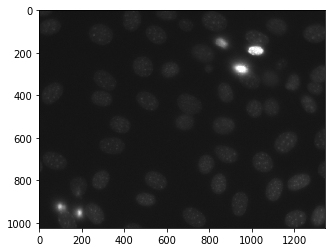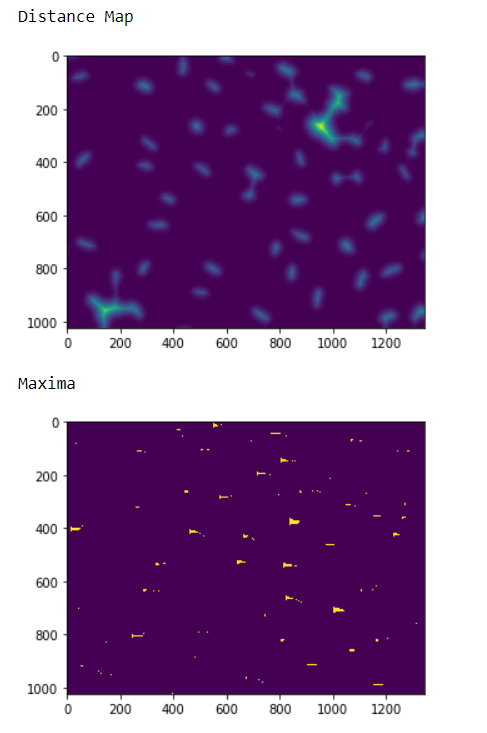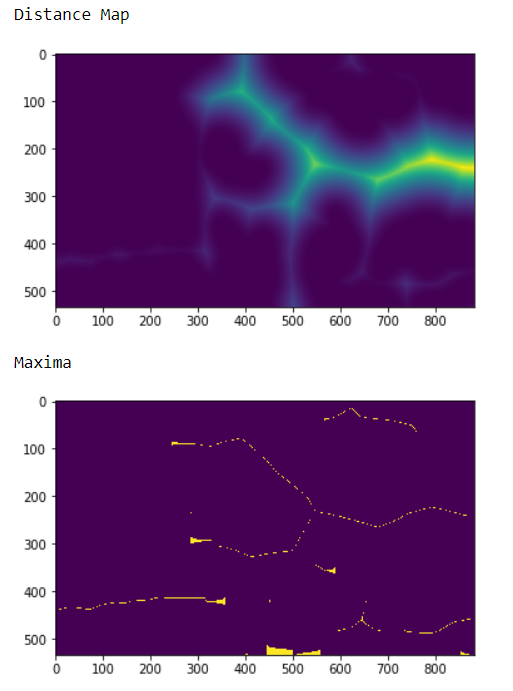Open in App
Not now

# Mahotas – Highlighting Image Maxima

• Last Updated : 17 May, 2022

In this article we will see how we can highlight the maxima of image in mahotas. Maxima can be best found in the distance map image because in labeled image each label is maxima but in distance map maxima can be identified easily. For this we are going to use the fluorescent microscopy image from a nuclear segmentation benchmark. We can get the image with the help of command given below

`mahotas.demos.nuclear_image()`

Below is the nuclear_imageIn order to do this we will use mahotas.morph.regmax method

Syntax : mahotas.morph.regmax(img, Bc)
Argument : It takes image object and numpy ones array as argument
Return : It returns image object

Note : The input of  this should  be the filtered image or loaded as grey
In order to filter the image we will take the image object which is numpy.ndarray and filter it with the help of indexing, below is the command to do this

`image = image[:, :, 0]`

Example 1 :

## Python3

 `# importing various libraries``import` `mahotas``import` `mahotas.demos``import` `mahotas as mh``import` `numpy as np``from` `pylab ``import` `imshow, show` `# loading nuclear image``nuclear ``=` `mahotas.demos.nuclear_image()` `# filtering image``nuclear ``=` `nuclear[:, :, ``0``]` `# adding gaussian filter``nuclear ``=` `mahotas.gaussian_filter(nuclear, ``4``)` `# setting threshold``threshed ``=` `(nuclear > nuclear.mean())` `# creating distance map``dmap ``=` `mahotas.distance(threshed)` `print``(``"Distance Map"``)``# showing image``imshow(dmap)``show()` `# numpy ones array``Bc ``=` `np.ones((``3``, ``2``))` `# getting maxima``maxima ``=` `mahotas.morph.regmax(dmap, Bc ``=` `Bc)` `# showing image``print``(``"Maxima"``)``imshow(maxima)``show()`

Output :Example 2 :

## Python3

 `# importing required libraries``import` `numpy as np``import` `mahotas``from` `pylab ``import` `imshow, show`` ` `# loading image``img ``=` `mahotas.imread(``'dog_image.png'``)``   ` `# filtering the image``img ``=` `img[:, :, ``0``]``    ` `# setting gaussian filter``gaussian ``=` `mahotas.gaussian_filter(img, ``15``)`` ` `# setting threshold value``gaussian ``=` `(gaussian > gaussian.mean())`` ` `# creating a labelled image``labelled, n_nucleus ``=` `mahotas.label(gaussian)`` `  `# getting distance map``dmap ``=` `mahotas.distance(labelled)` `# showing image``print``(``"Distance Map"``)``imshow(dmap)``show()`  `# numpy ones array``Bc ``=` `np.ones((``4``, ``1``))` `# getting maxima``maxima ``=` `mahotas.morph.regmax(dmap, Bc ``=` `Bc)` `# showing image``print``(``"Maxima"``)``imshow(maxima)``show()`

Output :My Personal Notes arrow_drop_up